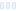Pink Grapefruit Energy Formula

G Fuel

Main info:

Pink Grapefruit Energy Formula
G Fuel
1 scoop
25.0 Calories
5.0 g
0 g
0 g

0 g
0 mg
0 g
79.0 mg
0 g
0 g

Percent calories from...
Nutrition Facts
For a Serving Size of (g)
How many calories are in Pink Grapefruit Energy Formula? Amount of calories in Pink Grapefruit Energy Formula: Calories Calories from Fat (%)
% Daily Value *
How much fat is in Pink Grapefruit Energy Formula? Amount of fat in Pink Grapefruit Energy Formula: Total Fat
How much sodium is in Pink Grapefruit Energy Formula? Amount of sodium in Pink Grapefruit Energy Formula: Sodium
How many carbs are in Pink Grapefruit Energy Formula? Amount of carbs in Pink Grapefruit Energy Formula: Carbohydrates
How many net carbs are in Pink Grapefruit Energy Formula? Amount of net carbs in Pink Grapefruit Energy Formula: Net carbs
How much sugar is in Pink Grapefruit Energy Formula? Amount of sugar in Pink Grapefruit Energy Formula: Sugar
How much protein is in Pink Grapefruit Energy Formula? Amount of protein in Pink Grapefruit Energy Formula: Protein
Vitamins and minerals
How much Calcium is in Pink Grapefruit Energy Formula? Amount of Calcium in Pink Grapefruit Energy Formula: Calcium
How much Iron is in Pink Grapefruit Energy Formula? Amount of Iron in Pink Grapefruit Energy Formula: Iron
Fatty acids
Amino acids
* The Percent Daily Values are based on a 2,000 calorie diet, so your values may change depending on your calorie needs.Loading similar foods...
Note: Any items purchased after clicking our Amazon buttons will give us a little referral bonus. If you do click them, thank you!Be cool

- Zen orangeI never skip arm day

- Buff broccoli